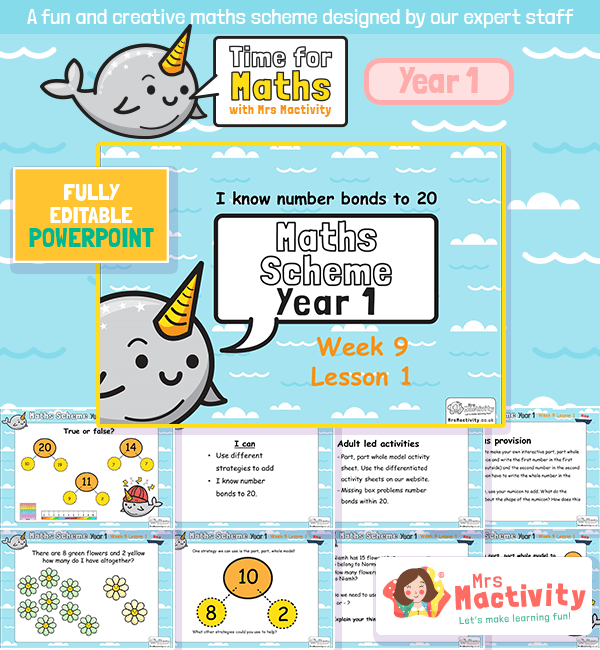# Number Bonds to 20 Maths PowerPointNew! Time for maths – the new maths scheme of work by Mrs Mactivity! Our maths scheme of work consists of daily PowerPoints with accompanying teacher notes and resources that can be easily used by teachers who want to save time and delivery interesting maths lessons that help children make progress. Our maths scheme centres around a maths mastery approach with plenty of opportunities to develop fluency and confidence in maths.

This particular lesson – Number Bonds to 10 Maths PowerPoint Year 1 Maths Scheme Week 9 Lesson 1 – covers the following National Curriculum objectives:

• represent and use number bonds and related subtraction facts within 20.
• read, write and interpret mathematical statements involving addition (+), subtraction (–) and equals (=) signs
• add and subtract one-digit and two-digit numbers to 20, including zero
• solve one-step problems that involve addition and subtraction, using concrete objects and pictorial representations, and missing number problems such as 7 = ☐ – 9

Explore our year 1 maths resources.

Explore our maths scheme.

Explore our addition and subtraction resources.

## Recently Viewed